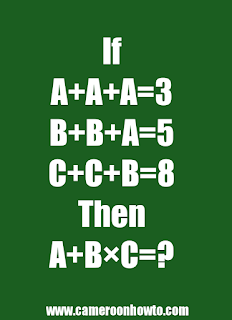0

# alphabet math puzzle A+B×C=? answer# alphabet math puzzle A+B×C=? answer

This alphabet math puzzle is confusing many people on the internet. When I posted it on my Facebook page, Many people failed it. In fact about 80% of those who attempted it had wrong answers. Today I will solve it for you in detail.

Here's the puzzle: if

A+A+A=3

B+B+A=5

C+C+B=8

Then

A+B×C=?. The correct answer would be 7 not 9, check details below.

Many people solve math quiz, puzzles to relax their mind. This one is simple and tricky. Make sure to test your friends, family members  and loved ones with the Puzzle. Also read

To solve this kind of Puzzle, you need to apply common sense, use the simultaneous equation method and apply BODMAS at the end. That's the trick in it.

So, first equation: A+A+A=3

Implies 3A = 3

Implies A=3÷3

Therefore A = 1

Second equation:B+B+A=5

B+B+1= 5 ( value of A =1 from equation 1).

2B = 5-1

B = 2.

Third equation:C+C+B=8

2C +2 = 8

2C = 8-2

C = 3.

Therefore the value of A= 1

B = 2

C = 3.

I will substitute the values above in the final equation.

Final equation: A+B×C=?

Implies 1+2×3 =?

Implies 1+ 6 =?(Apply BODMAS)

Implies 7 =?.

Therefore the correct answer to A+A+A=3

B+B+A=5

C+C+B=8

A+B×C=?, Is 7.

Some people will take 1+2= 3, then 3×3= 9 which is wrong.